The Art of Interface

# Mean filter, or average filter

Category. Digital signal and image processing (DSP and DIP) software development.

Abstract. The article is a practical guide for mean filter, or average filter understanding and implementation. Article contains theory, C++ source code, programming instructions and sample application.

Reference. Adaptive averaging technique: diffusion filter.## 1. Introduction to mean filter, or average filter

Mean filter, or average filter is windowed filter of linear class, that smoothes signal (image). The filter works as low-pass one. The basic idea behind filter is for any element of the signal (image) take an average across its neighborhood. To understand how that is made in practice, let us start with window idea.

## 2. Filter window or mask

Let us imagine, you should read a letter and what you see in text restricted by hole in special stencil like this.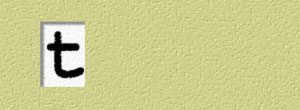Fig. 1. First stencil.

So, the result of reading is sound [t]. Ok, let us read the letter again, but with the help of another stencil: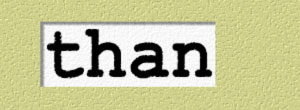Fig. 2. Second stencil.

Now the result of reading t is sound [ð]. Let us make the third try: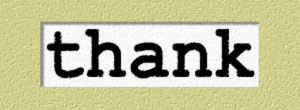Fig. 3. Third stencil.

Now you are reading letter t as sound [θ].

What happens here? To say that in mathematical language, you are making an operation (reading) over element (letter t). And the result (sound) depends on the element neighborhood (letters next to t).

And that stencil, which helps to pick up element neighborhood, is window! Yes, window is just a stencil or pattern, by means of which you are selecting the element neighborhood — a set of elements around the given one — to help you make decision. Another name for filter window is mask — mask is a stencil, which hides elements we are not paying attention to.

In our example the element we are operating on positioned at leftmost of the window, in practice however its usual position is the center of the window.

Let us see some window examples. In one dimension.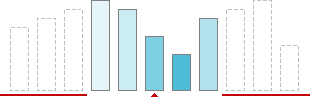Fig. 4. Window or mask of size 5 in 1D.

In two dimensions.Fig. 5. Window or mask of size 3×3 in 2D.

In three dimensions... Think about building. And now — about room in that building. The room is like 3D window, which cuts out some subspace from the entire space of the building. You can find 3D window in volume (voxel) image processing.Fig. 6. Window or mask of size 3×3×3 in 3D.

## 3. Understanding mean filter

Now let us see, how to “take an average across element's neighborhood”. The formula is simple — sum up elements and divide the sum by the number of elements. For instance, let us calculate an average for the case, depicted in fig. 7.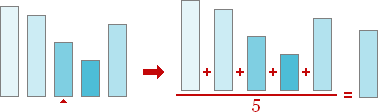Fig. 7. Taking an average.

And that is all. Yes, we just have filtered 1D signal by mean filter! Let us make resume and write down step-by-step instructions for processing by mean filter.

Mean filter, or average filter algorithm:

1. Place a window over element;
2. Take an average — sum up elements and divide the sum by the number of elements.

Now, when we have the algorithm, it is time to write some code — let us come down to programming.

## 4. 1D mean filter programming

In this section we develop 1D mean filter with window of size 5. Let us have 1D signal of length N as input. The first step is window placing — we do that by changing index of the leading element:

``````//   Move window through all elements of the signal
for (int i = 2; i < N - 2; ++i)``````

Pay attention, that we are starting with the third element and finishing with the last but two. The problem is we cannot start with the first element, because in this case the left part of the filter window is empty. We will discuss below, how to solve that problem.

The second step is taking the average, ok:

``````//   Take the average
result[i - 2] = (
signal[i - 2] +
signal[i - 1] +
signal[i] +
signal[i + 1] +
signal[i + 2]) / 5;``````

Now, let us write down the algorithm as function:

``````//   1D MEAN FILTER implementation
//     signal - input signal
//     result - output signal
//     N      - length of the signal
void _meanfilter(const element* signal, element* result, int N)
{
//   Move window through all elements of the signal
for (int i = 2; i < N - 2; ++i)
//   Take the average
result[i - 2] = (
signal[i - 2] +
signal[i - 1] +
signal[i] +
signal[i + 1] +
signal[i + 2]) / 5;
}``````

Type `element` could be defined as:

``typedef double element;``

## 5. Treating edges

For all window filters there is some problem. That is edge treating. If you place window over first (last) element, the left (right) part of the window will be empty. To fill the gap, signal should be extended. For mean filter there is good idea to extend signal or image symmetrically, like this:Fig. 8. Signal extension.

So, before passing signal to our mean filter function the signal should be extended. Let us write down the wrapper, which makes all preparations.

``````//   1D MEAN FILTER wrapper
//     signal - input signal
//     result - output signal
//     N      - length of the signal
void meanfilter(element* signal, element* result, int N)
{
//   Check arguments
if (!signal || N < 1)
return;
//   Treat special case N = 1
if (N == 1)
{
if (result)
result = signal;
return;
}
//   Allocate memory for signal extension
element* extension = new element[N + 4];
//   Check memory allocation
if (!extension)
return;
//   Create signal extension
memcpy(extension + 2, signal, N * sizeof(element));
for (int i = 0; i < 2; ++i)
{
extension[i] = signal[1 - i];
extension[N + 2 + i] = signal[N - 1 - i];
}
//   Call mean filter implementation
_meanfilter(extension, result ? result : signal, N + 4);
//   Free memory
delete[] extension;
}``````

As you can see, our code takes into account some practical issues. First of all we check our input parameters — signal should not be NULL, and signal length should be positive:

``````//   Check arguments
if (!signal || N < 1)
return;``````

Second step — we check case N=1. This case is special one, because to build extension we need at least two elements. For the signal of 1 element length the result is the signal itself. As well pay attention, our mean filter works in-place, if output parameter `result` is NULL.

``````//   Treat special case N = 1
if (N == 1)
{
if (result)
result = signal;
return;
}``````

Now let us allocate memory for signal extension.

``````//   Allocate memory for signal extension
element* extension = new element[N + 4];``````

And check memory allocation.

``````//   Check memory allocation
if (!extension)
return;``````

Now we are building extension.

``````//   Create signal extension
memcpy(extension + 2, signal, N * sizeof(element));
for (int i = 0; i < 2; ++i)
{
extension[i] = signal[1 - i];
extension[N + 2 + i] = signal[N - 1 - i];
}``````

Finally, everything is ready — filtering!

``````//   Call mean filter implementation
_meanfilter(extension, result ? result : signal, N + 4);``````

And to complete the job — free memory.

``````//   Free memory
delete[] extension;``````

Since we are using memory management function from standard library, we should include its header.

``#include <memory.h>``

## 6. 2D mean filter programming

In 2D case we have 2D signal, or image. The idea is the same, just now mean filter has 2D window. Window influences only the elements selection. The rest is the same: summing up the elements and dividing by their number. So, let us have a look at 2D mean filter programming. For 2D case we choose window of size 3×3.

``````//   2D MEAN FILTER implementation
//     image  - input image
//     result - output image
//     N      - width of the image
//     M      - height of the image
void _meanfilter(const element* image, element* result, int N, int M)
{
//   Move window through all elements of the image
for (int m = 1; m < M - 1; ++m)
for (int n = 1; n < N - 1; ++n)
//   Take the average
result[(m - 1) * (N - 2) + n - 1] = (
image[(m - 1) * N + n - 1] +
image[(m - 1) * N + n] +
image[(m - 1) * N + n + 1] +
image[m * N + n - 1] +
image[m * N + n] +
image[m * N + n + 1] +
image[(m + 1) * N + n - 1] +
image[(m + 1) * N + n] +
image[(m + 1) * N + n + 1]) / 9;
}``````

## 7. Treating edges in 2D case

As in 1D case in 2D case we should extend our input image as well. To do that we are to add lines at the top and at the bottom of the image and add columns to the left and to the right.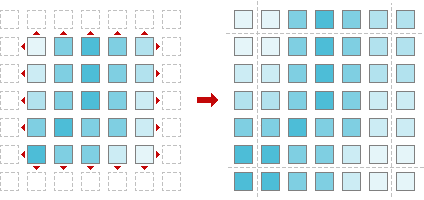Fig. 9. Image extension.

Here is our wrapper function, which does that job.

``````//   2D MEAN FILTER wrapper
//     image  - input image
//     result - output image
//     N      - width of the image
//     M      - height of the image
void meanfilter(element* image, element* result, int N, int M)
{
//   Check arguments
if (!image || N < 1 || M < 1)
return;
//   Allocate memory for signal extension
element* extension = new element[(N + 2) * (M + 2)];
//   Check memory allocation
if (!extension)
return;
//   Create image extension
for (int i = 0; i < M; ++i)
{
memcpy(extension + (N + 2) * (i + 1) + 1,
image + N * i,
N * sizeof(element));
extension[(N + 2) * (i + 1)] = image[N * i];
extension[(N + 2) * (i + 2) - 1] = image[N * (i + 1) - 1];
}
//   Fill first line of image extension
memcpy(extension,
extension + N + 2,
(N + 2) * sizeof(element));
//   Fill last line of image extension
memcpy(extension + (N + 2) * (M + 1),
extension + (N + 2) * M,
(N + 2) * sizeof(element));
//   Call mean filter implementation
_meanfilter(extension, result ? result : image, N + 2, M + 2);
//   Free memory
delete[] extension;
}``````

## 8. Mean filter library

Now we have four functions, two of them are for processing 1D signals by mean filter, and other two are for filtering 2D images. It is time to put everything together and create small mean filter library. Let us write code for header file.

``````#ifndef _MEANFILTER_H_
#define _MEANFILTER_H_

//   Signal/image element type
typedef double element;

//   1D MEAN FILTER, window size 5
//     signal - input signal
//     result - output signal, NULL for inplace processing
//     N      - length of the signal
void meanfilter(element* signal, element* result, int N);

//   2D MEAN FILTER, window size 3x3
//     image  - input image
//     result - output image, NULL for inplace processing
//     N      - width of the image
//     M      - height of the image
void meanfilter(element* image, element* result, int N, int M);

#endif``````

Our library is ready. The code we have written is good both for Linux/Unix and Windows platforms. You can download full mean filter library source code here:

Full listings of library files are available online as well:

And now — an application to play around!

## 9. Color mean filter: image smoothing, or blurring

We have created an application to demonstrate mean filter properties. The sample package includes 3 files — the application, sample image and description:

• smoother.exe — mean filter
• sample.bmp — 24-bit sample image
• readme.txt — description

Be aware of the fact, that this sample uses OpenGL, so it should be installed on your computer.

## 10. How to use

Start up smoother.exe application. Load the image.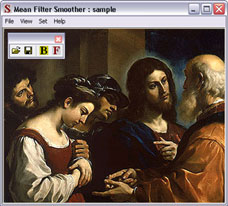Fig. 10. Original image.

Apply mean filter by choosing Set >> Filter or clicking F-button in toolbar. See the result. If necessary, filter the image one more time.Fig. 11. Image averaged with mean filter.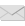Write to the author of the article — Sergey Chernenko